# Test: Digital Electronics- 1

## 15 Questions MCQ Test GATE ECE (Electronics) 2023 Mock Test Series | Test: Digital Electronics- 1

Description
Attempt Test: Digital Electronics- 1 | 15 questions in 45 minutes | Mock test for GATE preparation | Free important questions MCQ to study GATE ECE (Electronics) 2023 Mock Test Series for GATE Exam | Download free PDF with solutions
QUESTION: 1

### What are the essential prime implicants of following Boolean function x'z + y`z + xz'

Solution:

flx, y, z)= xlz + ylz + xzl These pairs are essential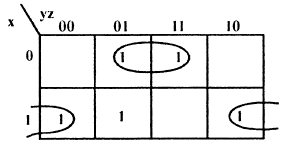QUESTION: 2

### Consider a multiplexer with X &Y as data inputs and z as a control pin. If Z = 0, selects input X and Z = I , selects input Y what are the connections required to realize the 2 - variable Boolean functions f T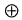R

Solution: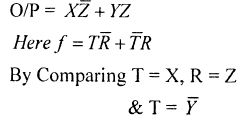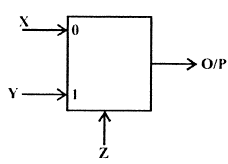QUESTION: 3

### A combinational circuit, using 8 x 1 MUX is shown in figure. The minimized expression for output Z is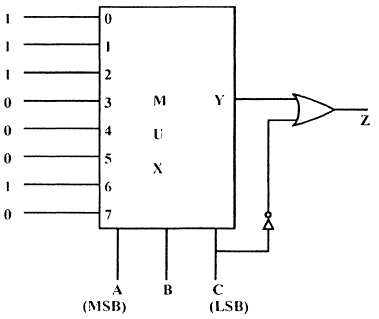Solution: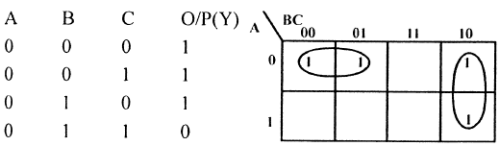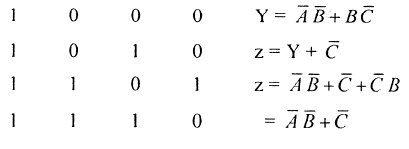QUESTION: 4

The Minimal Product of sums function described by k-Map, shown in figure is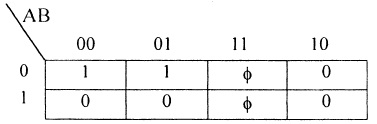Solution: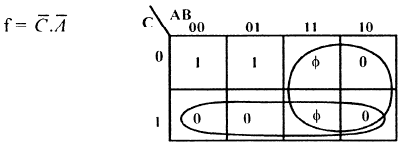QUESTION: 5

The output of an AND gate with three inputs, A, B, and C, is HIGH when ________.

Solution:
QUESTION: 6

The digital circuit using two inverters shown in figure will acts as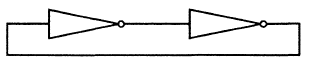Solution:

When 2 inverters are connected then it will become bi-stable.

QUESTION: 7

Why is a demultiplexer called a data distributor?

Solution:

A demultiplexer sends a single input to multiple outputs, depending on the select lines. For one input, the demultiplexer gives several outputs. That is why it is called a data distributor.

*Answer can only contain numeric values
QUESTION: 8

If 2's complement representation of a 16-bit number (one-sign bit and 15 Magnitude bits) is FFFFthen its magnitude in decimal representation is ____

Solution: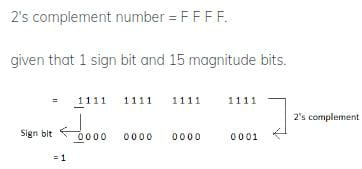QUESTION: 9

The initial state of MOD — 16, down counter is 0110. What state will be it be after 37 clock pulses??

Solution: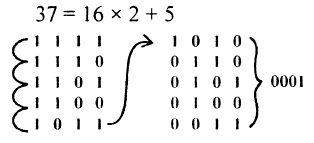QUESTION: 10

In the given figure shows a negative logic AND gate. If positive logic is used this gate is equivalent to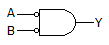Solution:

Y = A B = A + B .

QUESTION: 11

An 8085 Microprocessor based system uses a 4 k x 8 bit RAM whose starting address is AAOOH. The address of last byte in this RAM is

Solution:

4K x 8 = 212 x 8 bit it has 12 bits So address of last - byte will be AA 00 + 0 FFF = B9FF

QUESTION: 12

What is F's complement of (2BFD)16

Solution:

F'S complement of 2BFD is FFF F - 2BFD = D402

QUESTION: 13

A. ROM is interfaced to an 8085 CPU as indicated in figure, shown, what is address range occupied by ROM is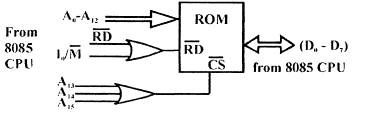Solution:

A15 A 14 A 13 will be 000 So Range will be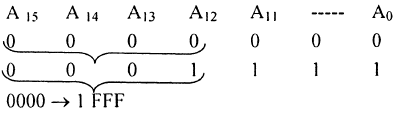QUESTION: 14

112 4-bit 2's complement no's 1011 and 0110 are added, then result expressed in 2's complementnotation is

Solution:
QUESTION: 15

Which one of the following statement is correct?

Solution:Use Code STAYHOME200 and get INR 200 additional OFF Use Coupon Code